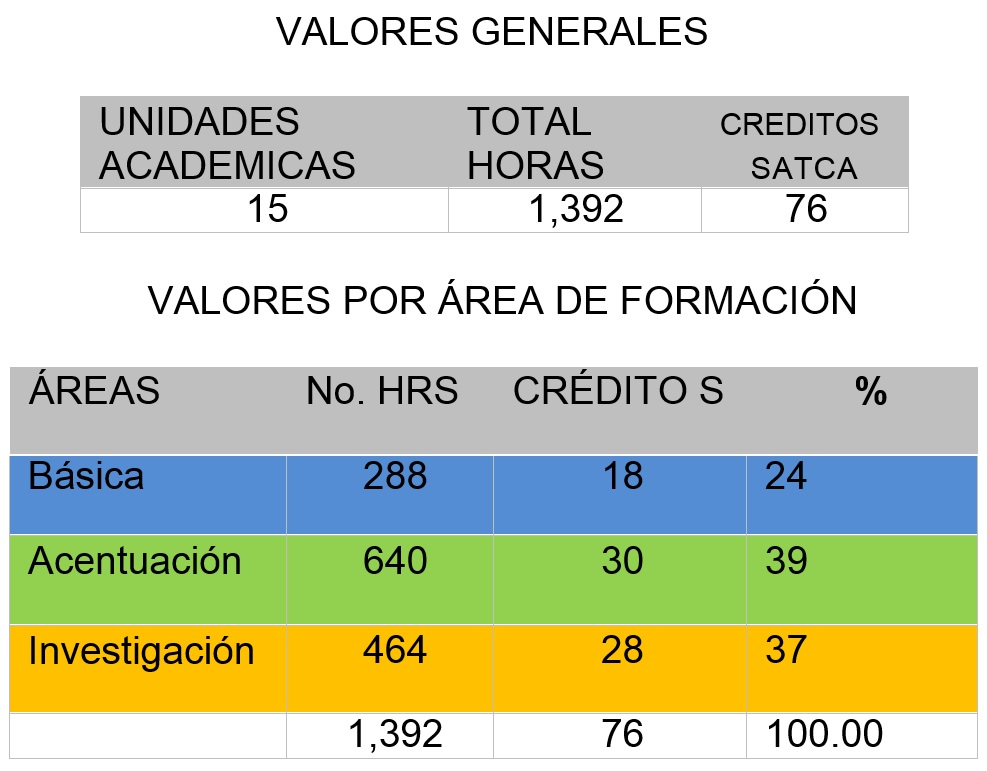# Organización y estructura curricular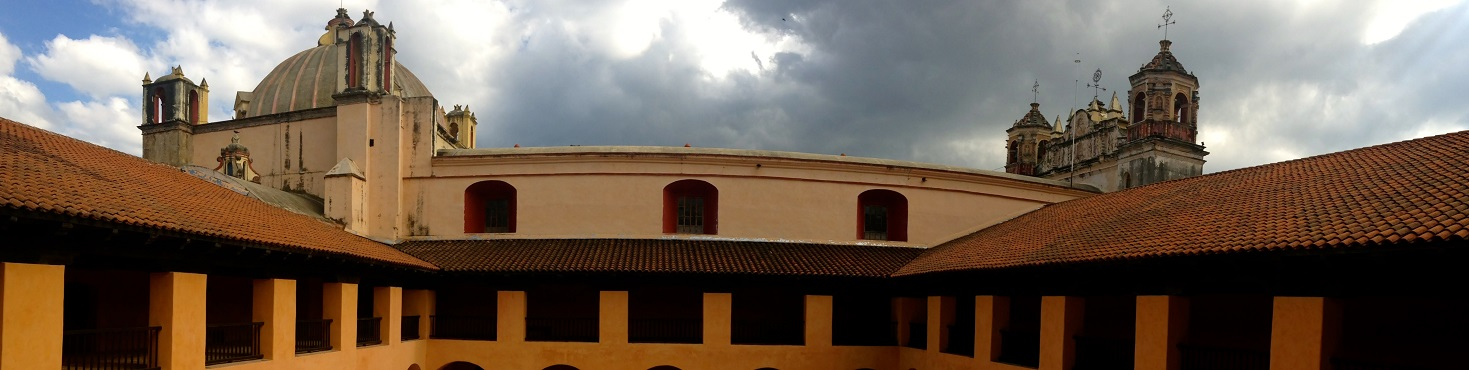O R G A N I Z A C I Ó N   Y   E S T R U C T U R A    C U R R Í C U L A R

 M A P A   C U R R Í C U L A R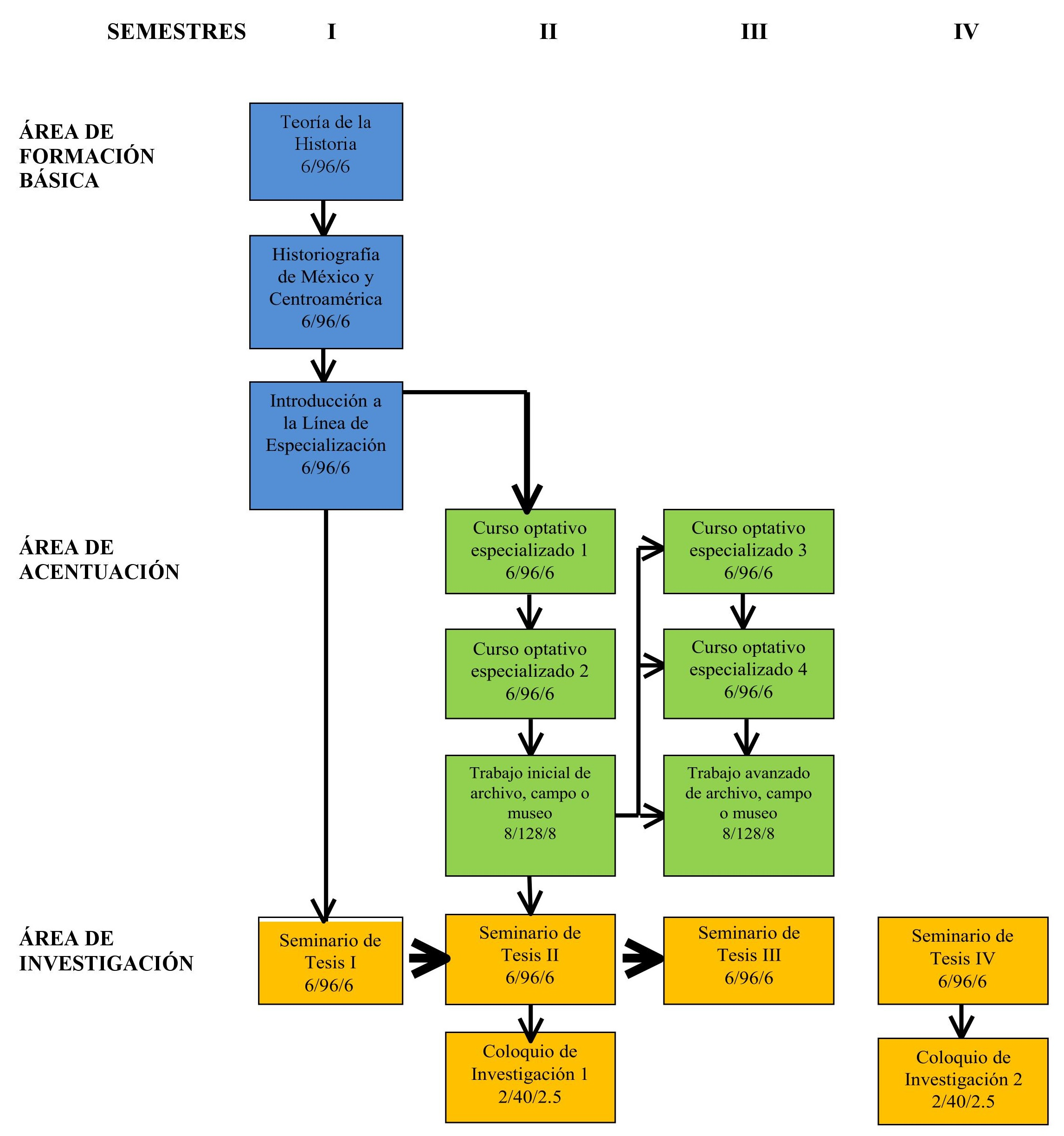P R I M E R   S E M E S T R E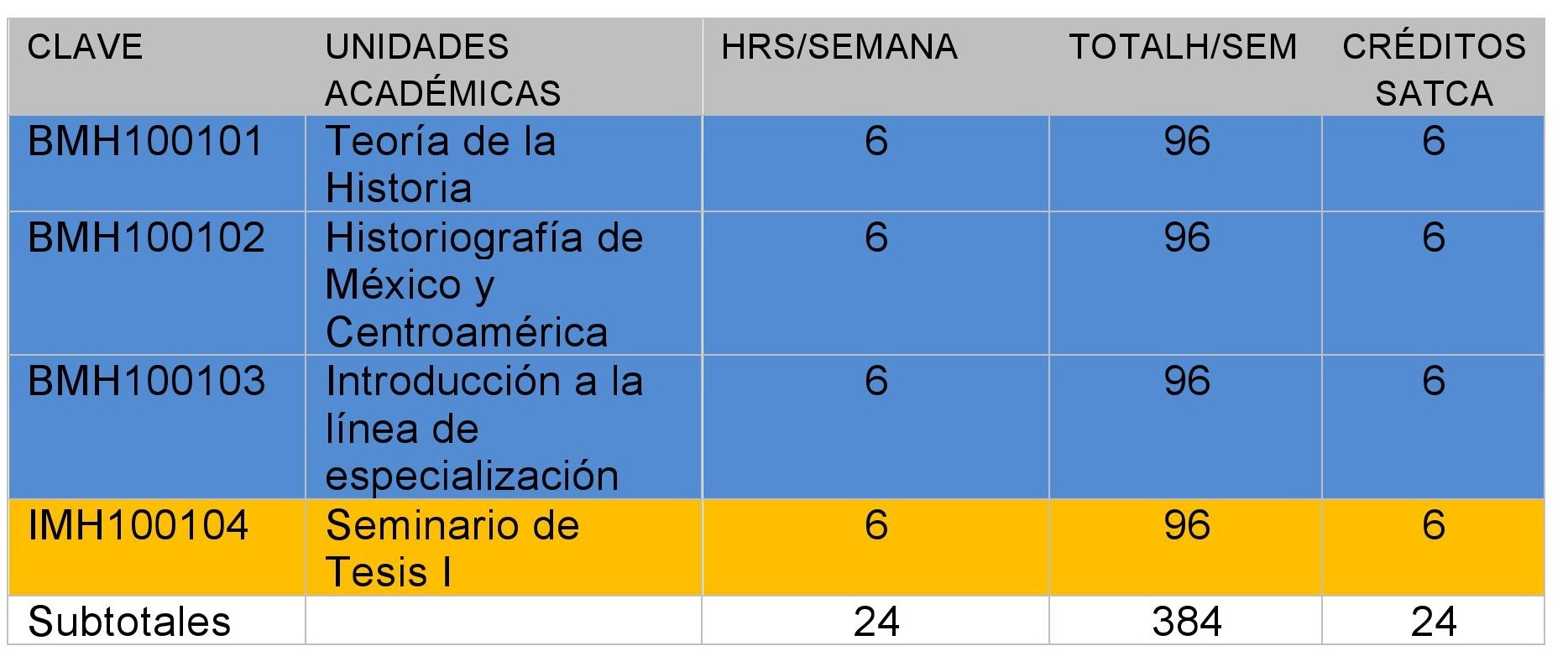S E G U N D O   S E M E S T R E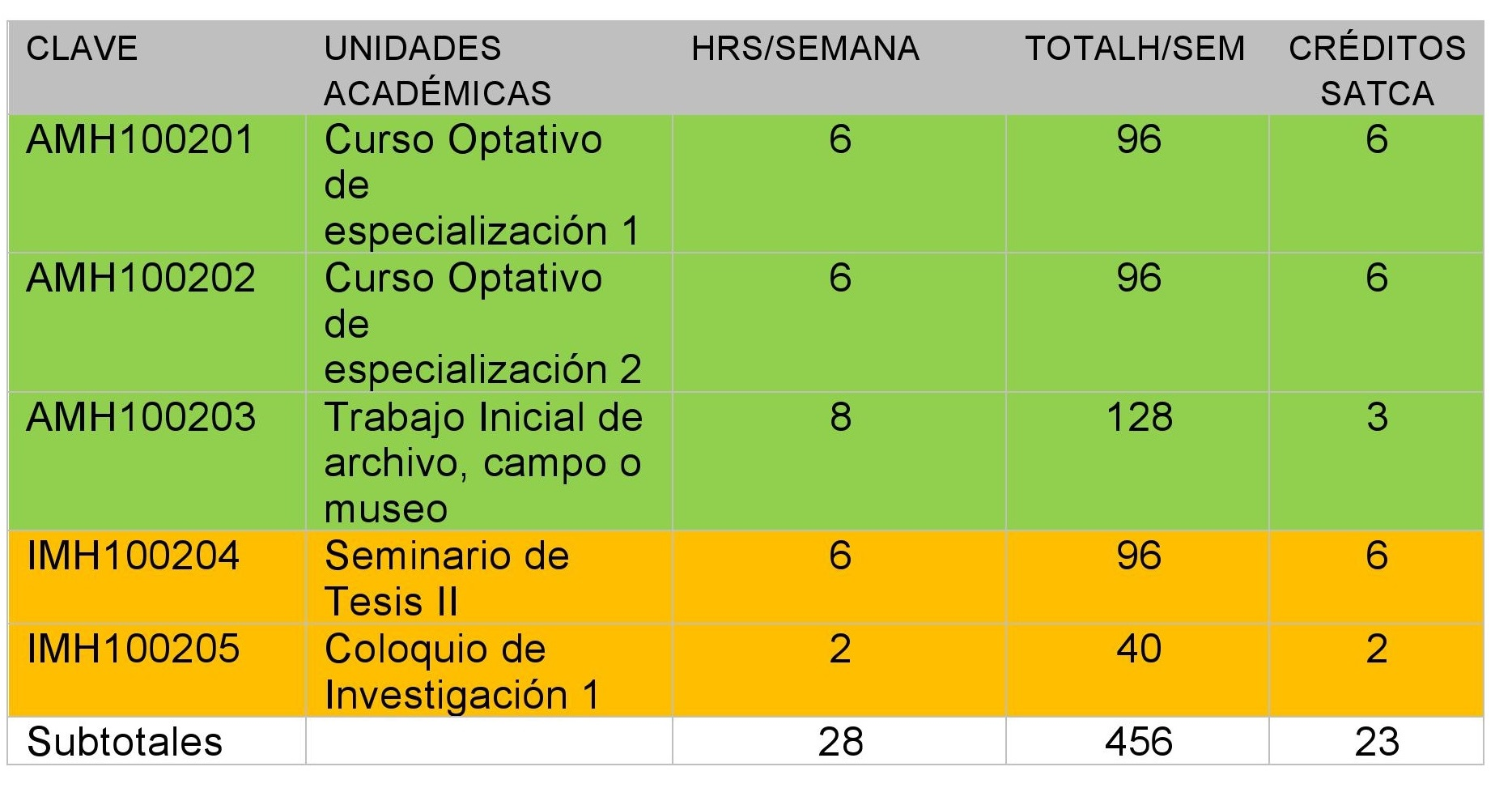T E R C E R   S E M E S T R E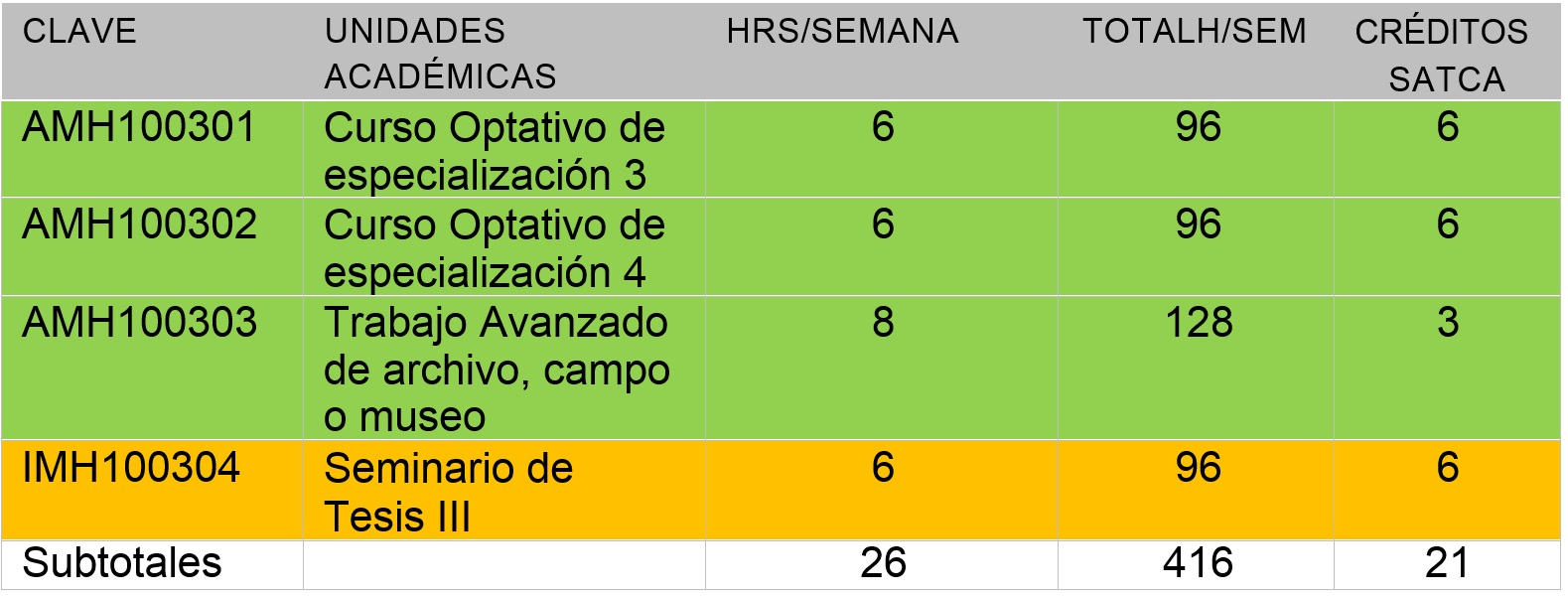C U A R T O    S E M E S T R E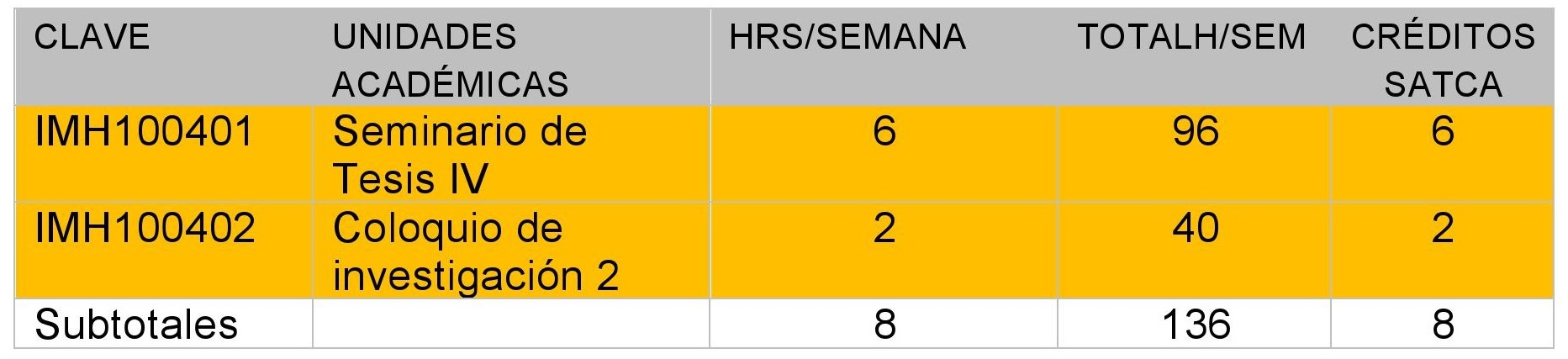T O T A L    D E    H O R A S    Y    C R É D I T O S